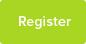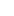# Tutor Hunt Questions

You have counted a suspension of yeast cells and calculate that you have to dilute your sample 1 plus 3.
If the yeast suspension is 7.3 x 107 cells/ml, you have a 100 ml sample and you need 30 ml of the diluted sample, how many ml of the yeast suspension do you pipette into your flask?

Express your answer to 2 places of decimals.
Thank you
3 years ago

Maths Question asked by Holly

Please enter your response to the question below. The student will get a notification as soon your response has been approved by our moderation team.

Here 1 + 3 means for our 100ml of yeast we need 3 * 100ml of water.

Adding 3 parts we have
100ml (yeast) + 300ml (water) = 400ml (diluted)......

Then we need to get 30ml of solution.

To get 30 ml there are 2 steps .....

1. We divide the 400ml by 400 to get 1ml.

400ml/400 = 1ml. .....

2. I multiply the 1ml by 30 to get 30ml.

1ml * 30 = 30ml....

Step 1 plus Step 2 we divide by 400 then multiply by 30.

400ml -> 30ml...

We can also write the 400ml as the 300ml of water plus 100ml of yeast and track what happens. Divide the parts by 400 and multiply them by 30 just like before. ......

300ml (w) + 100ml (y) -> (divide by 400) -> 0.75ml + 0.25ml ....
0.75ml + 0.25ml -> (multiply by 30) -> 22.5ml (w) + 7.5ml (y)

Which we can see adds together (22.5 + 7.5 = 30) to make our 30ml! The 30ml has 7.5ml of yeast in it!

So the answer is
7.50 ml of yeast (to 2 d.p.)

....
(To help, imagine we split a basket of 10 pears and 10 apples down the middle in half. We get half the total fruits which is made of half of the pears + half of the apples.
....
Eg. 20 fruits -> (half) -> 10 fruits.
10 pears + 10 apples -> (half) -> 5 pears + 5 apples. )